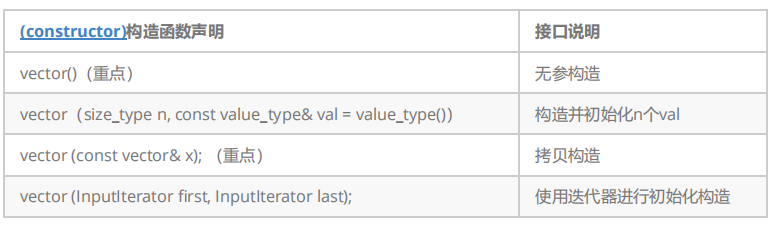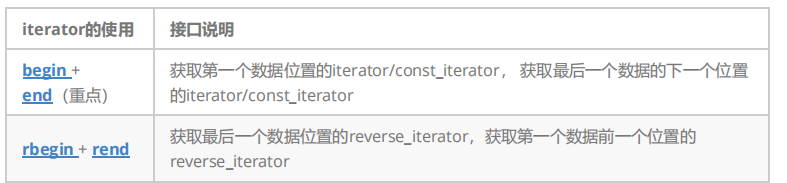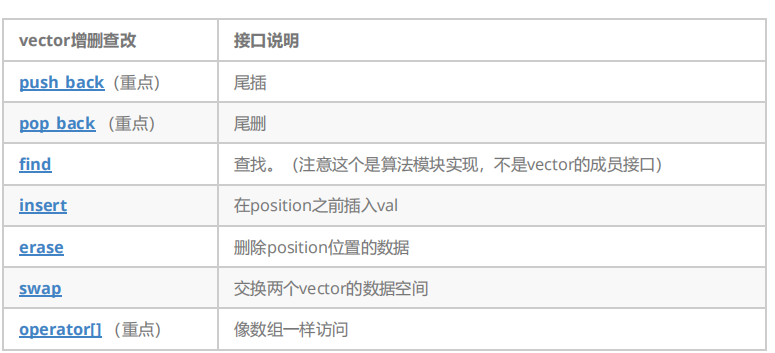# vector

vector的介绍

1. vector是表示可变大小数组的序列容器。

2. 就像数组一样，vector也采用的连续存储空间来存储元素。也就是意味着可以采用下标对vector的元素进行访问，和数组一样高效。但是又不像数组，它的大小是可以动态改变的，而且它的大小会被容器自动处理。

3. 本质讲，vector使用动态分配数组来存储它的元素。当新元素插入时候，这个数组需要被重新分配大小为了增加存储空间。其做法是，分配一个新的数组，然后将全部元素移到这个数组。就时间而言，这是一个相对代价高的任务，因为每当一个新的元素加入到容器的时候，vector并不会每次都重新分配大小。

4. 与其它动态序列容器相比（deques, lists and forward_lists）， vector在访问元素的时候更加高效，在末尾添加和删除元素相对高效。对于其它不在末尾的删除和插入操作，效率更低。

vector的使用vector iterator 的使用#include <iostream>
#include <vector>
using namespace std;

void PrintVector(const vector<int>& v) {
// const对象使用const迭代器进行遍历打印
vector<int>::const_iterator it = v.begin();
while (it != v.end())
{
cout << *it << " ";
++it;
}
cout << endl;
}
int main()
{
// 使用push_back插入4个数据
vector<int> v;
v.push_back(1);
v.push_back(2);
v.push_back(3);
v.push_back(4);
// 使用迭代器进行遍历打印
vector<int>::iterator it = v.begin();
while (it != v.end())
{
cout << *it << " ";
++it;
}
cout << endl;
// 使用迭代器进行修改
it = v.begin();
while (it != v.end())
{
*it *= 2;
++it;
}
// 使用反向迭代器进行遍历再打印
vector<int>::reverse_iterator rit = v.rbegin();
while (rit != v.rend())
{
cout << *rit << " ";
++rit;
}
cout << endl;
PrintVector(v);
return 0;
}


vector 增删查改vector 迭代器失效问题。（重点）

1.会引起其底层空间改变的操作，都有可能是迭代器失效，比如：resize、reserve、insert等。

#include <iostream>
using namespace std;
#include <vector>
int main()
{
vector<int> v{ 1,2,3,4,5,6 };

auto it = v.begin();

// 将有效元素个数增加到100个，多出的位置使用8填充，操作期间底层会扩容
// v.resize(100, 8);

// reserve的作用就是改变扩容大小但不改变有效元素个数，操作期间可能会引起底层容量改变
// v.reserve(100);

// 插入元素期间，可能会引起扩容，而导致原空间被释放
// v.insert(v.begin(), 0);
// v.push_back(8);

// 给vector重新赋值，可能会引起底层容量改变
v.assign(100, 8);

/*
出错原因：以上操作，都有可能会导致vector扩容，也就是说vector底层原理旧空间被释放掉，
而在打印时，it还使用的是释放之间的旧空间，在对it迭代器操作时，实际操作的是一块已经被释放的
空间，而引起代码运行时崩溃。
解决方式：在以上操作完成之后，如果想要继续通过迭代器操作vector中的元素，只需给it重新
赋值即可。
*/
while (it != v.end())
{
cout << *it << " ";
++it;
}
cout << endl;
return 0;
}


2.指定位置元素的删除操作–erase

#include <iostream>
using namespace std;
#include <vector>
int main()
{
int a[] = { 1, 2, 3, 4 };
vector<int> v(a, a + sizeof(a) / sizeof(int));
// 使用find查找3所在位置的iterator
vector<int>::iterator pos = find(v.begin(), v.end(), 3);
// 删除pos位置的数据，导致pos迭代器失效。
v.erase(pos);
cout << *pos << endl; // 此处会导致非法访问
return 0;
}

05-2817万+
03-17459702-0128万+
02-269万+
07-052268
08-296万+
05-175万+
07-292267